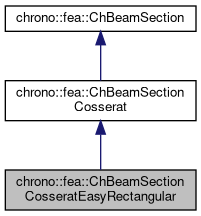chrono::fea::ChBeamSectionCosseratEasyRectangular Class Reference

## Description

A simple specialization of ChBeamSectionCosserat if you do not need to define its separate models for elasticity, plasticity, damping and inertia.

Good if you just need the simplest model for a rectangular centered beam. This section automatically creates, initializes and embeds, at construction, these models:

#include <ChBeamSectionCosserat.h>

Inheritance diagram for chrono::fea::ChBeamSectionCosseratEasyRectangular:[legend]
Collaboration diagram for chrono::fea::ChBeamSectionCosseratEasyRectangular:[legend]

## Public Member Functions

ChBeamSectionCosseratEasyRectangular (double width_y, double width_z, double E, double G, double density)Public Member Functions inherited from chrono::fea::ChBeamSectionCosserat
ChBeamSectionCosserat (std::shared_ptr< ChInertiaCosserat > minertia, std::shared_ptr< ChElasticityCosserat > melasticity, std::shared_ptr< ChPlasticityCosserat > mplasticity={}, std::shared_ptr< ChDampingCosserat > mdamping={})

virtual void ComputeStress (ChVector<> &stress_n, ChVector<> &stress_m, const ChVector<> &strain_e, const ChVector<> &strain_k, ChBeamMaterialInternalData *mdata_new=nullptr, const ChBeamMaterialInternalData *mdata=nullptr)
Compute the generalized cut force and cut torque, given the actual generalized section strain expressed as deformation vector e and curvature k, that is: {F,M}=f({e,k}), and given the actual material state required for plasticity if any (but if mdata=nullptr, computes only the elastic force). More...

virtual void ComputeStiffnessMatrix (ChMatrixNM< double, 6, 6 > &K, const ChVector<> &strain_e, const ChVector<> &strain_k, const ChBeamMaterialInternalData *mdata=nullptr)
Compute the 6x6 tangent material stiffness matrix [Km] = dσ/dε Compute the 6x6 tangent material stiffness matrix [Km] = dσ/dε at a given strain state, and at given internal data state (if mdata=nullptr, computes only the elastic tangent stiffenss, regardless of plasticity). More...

void SetElasticity (std::shared_ptr< ChElasticityCosserat > melasticity)
Set the elasticity model for this section. More...

std::shared_ptr< ChElasticityCosseratGetElasticity ()
Get the elasticity model for this section. More...

void SetPlasticity (std::shared_ptr< ChPlasticityCosserat > mplasticity)
Set the plasticity model for this section. More...

std::shared_ptr< ChPlasticityCosseratGetPlasticity ()
Get the elasticity model for this section, if any. More...

void SetInertia (std::shared_ptr< ChInertiaCosserat > minertia)
Set the inertial model for this section, that defines the mass per unit length and the inertia tensor of the section.

std::shared_ptr< ChInertiaCosseratGetInertia ()
Get the inertial model for this section, if any. More...

void SetDamping (std::shared_ptr< ChDampingCosserat > mdamping)
Set the damping model for this section. More...

std::shared_ptr< ChDampingCosseratGetDamping ()
Get the damping model for this section. More...Public Member Functions inherited from chrono::fea::ChBeamSection
void SetDrawShape (std::shared_ptr< ChBeamSectionShape > mshape)
Set the graphical representation for this section. More...

std::shared_ptr< ChBeamSectionShapeGetDrawShape () const
Get the drawing shape of this section (i.e.a 2D profile used for drawing 3D tesselation and visualization) By default a thin square section, use SetDrawShape() to change it.

void SetDrawThickness (double thickness_y, double thickness_z)
Shortcut: adds a ChBeamSectionShapeRectangular for visualization as a centered rectangular beam, and sets its width/height. More...

Shortcut: adds a ChBeamSectionShapeCircular for visualization as a centered circular beam, and sets its radius. More...

void SetCircular (bool ic)
OBSOLETE only for backward compability

## ◆ ChBeamSectionCosseratEasyRectangular()

 chrono::fea::ChBeamSectionCosseratEasyRectangular::ChBeamSectionCosseratEasyRectangular ( double width_y, double width_z, double E, double G, double density )
Parameters
 width_y width of section in y direction width_z width of section in z direction E Young modulus G shear modulus density volumetric density (ex. in SI units: [kg/m^3])

The documentation for this class was generated from the following files:
• /builds/uwsbel/chrono/src/chrono/fea/ChBeamSectionCosserat.h
• /builds/uwsbel/chrono/src/chrono/fea/ChBeamSectionCosserat.cpp